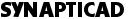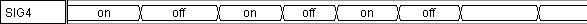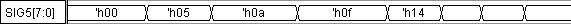# State Label Equations

State label equations provide an easy way to label signals with state patterns. You can label an entire signal with only one equation. State label equations are entered in the Signal Properties dialog, in the edit box next to the Label Eqn button. After entering the equation, press the Label Eqn button to apply it to the  signal.

State Label Equations Syntax rules:

State label equations are made up by combining the various label functions. Functions can be applied in sequence by listing them in a comma separated list. Each label function returns a value of list. Whenever you see list, you can use a function, a single value like "5" or "green", or a list in parentheses (2,3,4).

Inc(start,increment,count)

This function will generate labels that begin at "start" and increase by "increment" each time. A total of "count" labels will be generated.

Example: Inc(0,2,10) - Labels the signal with values 0,2,4,6,8,10,12,14,16,18Dec(start,increment,count)

This function will generate labels that begin at "start" and decrease by "increment" each time. It is equivalent to calling Inc(start,-increment,count).

Example: Rep(Dec(3,1,3),2) - Labels the signal with values 3,2,1,3,2,1Rep(list,count)

This function repeats list, count number of times. "The list" can either be a literal list - (1,3,4) or ("red","orange","yellow") for example,  or it can be a function that generates a list (Inc, Dec,or Rep).

Example: Rep(("on","off"),3) - Labels the signals with the values on,off,on,off,on,off.Note: If the list contains a text string, place it in quotes.

Hex(list)

This function formats the list as hexadecimal numbers. All numbers in the list are converted to a base-16 representation. The letters a-f are lowercase, and the numbers are prefixed by "`h" to indicate that they are hexadecimal. By default all numbers in the list are formatted to the same number of digits, adding leading zeros as necessary. If a MSB and LSB for the signal are specified in the Signal Properties dialog , all numbers will be formatted to display the given number of bits.

Example: Hex(Inc(0,5,5)) - Labels the signal with the values 'h0,'h5,'ha,'hf,'h14.Bin(list)

This function formats the list as binary. By default, all numbers in the list are the same length, with leading zeros as necessary. If a MSB and LSB for the signal are specified in the Signals Property dialog, all numbers will be formatted to display the given number of bits.

Example: Bin(Inc(0,3,5)) - Labels the signal with the values 0000,0011,0110,1001,1100Skip(integer)

This function format causes a number of segments to be skipped (left unchanged) by the equation.

Example: Skip(3),Inc(0,1,4),Dec(2,1,3) - Labels the signal with the values 0,1,2,3,2,1,0 starting at the fourth segment. The first three segments are skipped, leaving them with their original label (blank if they had no label to start with). Any number of functions can be chained together in this style, separating them with commas.Back to Application Notes